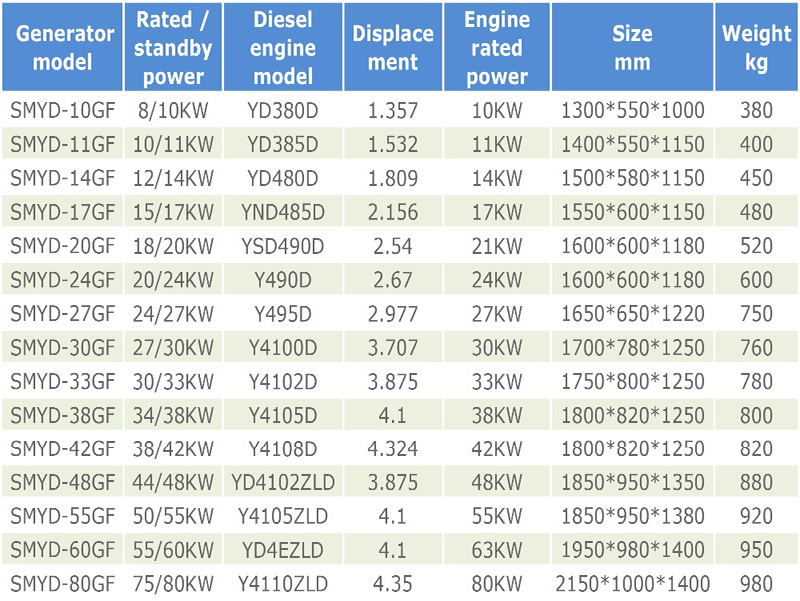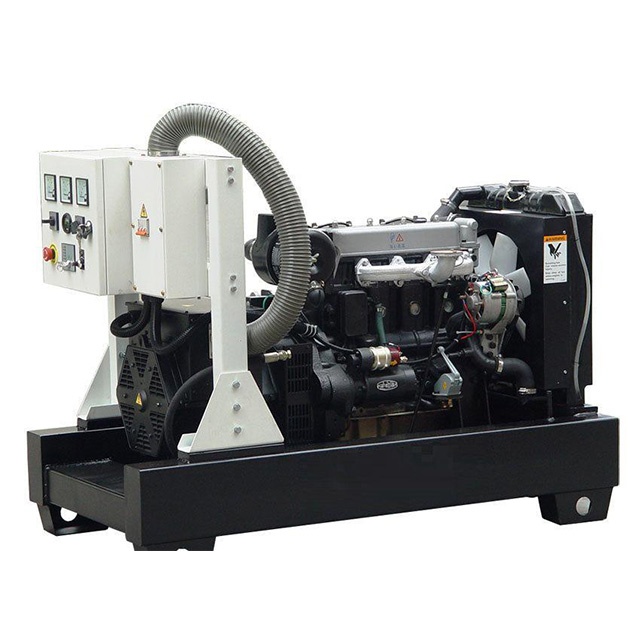# Causes of fouling in heap leaching - multiphase ion balance in solution

First, solubility

The solubility properties of different kinds of substances in the same solvent often vary widely. To characterize this difference in the substance, the term "solubility" is generally used. Solubility is different from concentration. Concentration refers to the relative content of solvent and solute in solution. Solubility refers to the relative content of solvent and solute in saturation and solution. Under a certain temperature and pressure, the content of solute in a certain amount of saturated solution is called solubility. It is customary to use 100 g of solvent to dissolve the maximum number of grams of solute to indicate solubility.

The solvent in the heap leaching is water, and the solubility of the substance in water is determined first by their nature, and secondly by the conditions of dissolution. The solubility of some substances influenced by temperature, the gas pressure is also affected by the influence of metal ions affected by pH value, the solubility of many substances by ionic strength of the solution, and the like.

Second, the solubility product and multiphase ion balance

It is known from the meaning of solubility that there is a limit to the dissolution of any insoluble electrolyte in water. When the ion concentration of the insoluble electrolyte in the solution reaches a certain value, crystallization or precipitation occurs. Taking the calcium carbonate scale which is common in gold heap leaching as an example, the Ca 2 + dissolved in the ore and the CO 3 2 - produced by the change of the pH value of the solution, when the concentration of both is supersaturated, the following reaction occurs. :

Ca 2 + +CO 3 2 -CaCO 3 â†“

According to the law of mass action, Ksp=[CO 3 2 - ][Ca 2 + ]

Ksp reflects the properties of such poorly soluble electrolyte compounds. This formula indicates that the dissolution and crystallization (or precipitation) balance of the poorly soluble electrolyte in aqueous solution depends on its ion concentration product in the aqueous solution. When the temperature is constant, the ion concentration product is a constant.

The solubility product is defined as the product of the concentration of each ion in the saturated solution under certain conditions, expressed as Ksp. If expressed by the general formula, then

AmBn (solid)mA n + +nB m -

Ksp=[A n + ] m [B m - ] n

The above reaction indicates that under certain conditions, the precipitation-dissolution equilibrium of the insoluble electrolyte AmBn in a saturated solution establishes a balance between solid and ions in the solution, which is called heterogeneous ion balance. We can use the solubility product rule to judge the reaction direction of multiphase ion balance:

When Ksp>[A n + ] m [B m - ] n , the solid is dissolved, and no precipitation or crystallization occurs in the solution;

When Ksp=[A n + ] m [B m - ] n , the solid in the solution is not dissolved, and no precipitation occurs, and the dissolution-precipitation equilibrium is reached;

When Ksp < [A n + ] m [B m - ] n , a precipitate is generated in the solution until [A n + ] m [B m - ] n = Ksp.

The relationship between the solubility of the insoluble electrolyte and the solubility product. For the same type of poorly soluble electrolytes, such as CaSO 4 and CaCO 3 , AgCl and AgBr, the smaller the Ksp, the smaller the solubility, and vice versa. However, this principle is not suitable for different types of poorly soluble electrolytes such as AgCl and Ag 2 CrO 4 .

According to the solubility product rule, in order to prevent the ions in the solution from forming crystals or precipitates, the solution should be in an unsaturated state, and the ion content in the solution should be reduced as much as possible so that the ion concentration product in the solution is smaller than the Ksp. In gold ore heap leaching, the use of caustic soda instead of lime as a protective base is to reduce the calcium ion content in the solution, and some to reduce the concentration of CO 3 2 - in the solution, and to control the pH of the solution to 9.5.

Third, the hydrolysis equilibrium of metal ions

In the acid heap leaching, the acidity is high in the upper part of the immersion reactor, and the acid and the ore react strongly. A large amount of metal ions in the ore, such as iron and aluminum , enter the solution. Due to the continuous reaction, the acid is gradually consumed, and the pH of the solution at the bottom of the immersion reactor It rises and the metal ions begin to precipitate. The essence of the hydrolysis of metal ions into scale is that the metal ions dissolved and leached in the leachate are precipitated by the metal hydroxide precipitate due to the increase in pH, and are represented by the following formula:

Me n + +nOH -Me(OH)n (A)

OH - derived from water dissociation

nH 2 OnH + +nOH - (B)

The general formula for the hydrolysis of metal ions by the formulas (A) and (B)

Me n + +nH 2 OnH + +Me(OH)n (C)

lgK=lg(D)

For any given metal ion hydrolysis reaction, lgK in (D) is a constant (assuming a certain temperature), and lgKâˆ•n is C, then

pH=C-()lg[Me n + ] (E)

It can be seen from the formula (E) that the concentration of the metal ion Me n + in the solution is closely related to the pH value.

Using the concept of solubility product, if we know the Ksp of the insoluble metal hydroxide and the concentration of the metal ion in the solution, we can calculate the pH at which precipitation occurs. For example, when the concentration of Fe 3 + in the solution is 0.1 mol âˆ•L, the equilibrium is determined by precipitation-dissolution.

Fe(OH) 3 (solid)Fe 3 + +3OH -

Ksp=[Fe 3 + ][OH - ] 3 =4Ã—10 -38

Then [OH - ]==7.4Ã—10 -13 molâˆ•L

[H + ]==1.35Ã—10 -2 molâˆ•L

pH=-lg(1.35Ã—10 -2 )=1.87â‰Œ1.9

(Kw is the dissociation constant of the bearing)

From this calculation, it was found that the initial hydrolysis pH of 0.1 mol of FeL Fe 3 + was 1.9. If the Fe 3 + precipitation is to be completed, even if the Fe 3 + concentration in the solution reaches 1 Ã— 10 -5 mol âˆ• L, its pH value can be calculated to be 3.2.

The following table shows the solubility products of some poorly soluble compounds and the pH of metal hydroxide precipitates associated with gold, uranium , and copper heap leaching.

### Our diesel generator factory is in Jiangsu Province, China, is a Factory with OEM service.Yangdong Diesel Generator

Yangdong Diesel Generator,Yangdong Power Generator,38Kw Yangdong Diesel Generator,25Kva Power Generator

Jiangsu Province Suanma Power Equipment Co., Ltd. , https://www.suanma-generator.com